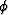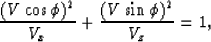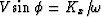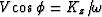Next: HORIZONTALLY LAYERED INVERSION Up: Cunha: anisotropic traveltime inversion Previous: Introduction

# THE HOMOGENEOUS INVERSION SCHEME

For elliptically anisotropic media, that is, media in which the group velocity V has an elliptical dependence on the angle of energy propagation, such that(1)
the relation between group and phase velocities and angles is given by a closed analytical form (Levin, 1978):(2) (3)
where v andare the phase velocity and angle, respectively. Equation ( 1) also represents an elliptical dispersion relation, sinceand.It turns out that elasticity theory predicts this elliptical dependence only for SH in a transverse isotropic symmetry, or for all wave types in the trivial case of isotropic material where the ellipse becomes a circle. However, the use of an elliptical approximation for the dispersion relation can considerably simplify the problem of estimating the elastic parameters from the recorded wavefields.

If we consider a source and a receiver separated by a vertical distance z and a horizontal distance x, the traveltime t predicted by equation (1) is(4)
The relation between the slownesses squared (or sloth) Mx and Mzthat best fits our data and the true vertical and horizontal sloths of the medium Mv and Mh respectively will depend on the specific geometry in which the data was collected. For an usual surface-seismic geometry, equation (4) correspond to a paraxial approximation around the vertical axis. In this case, Mx corresponds to the estimated normal-moveout (NMO) sloth, whileis the vertical traveltime. If z is unknown, then Mz is an arbitrary factor that cannot be estimated (Dellinger and Muir, 1985).

If both distances, x and z, are known it is possible to estimate both sloth parameters by fitting the data to equation (4). For surface seismic surveys the estimated Mz will be close to the vertical sloth Mv, whereas for a cross-well geometry the estimated Mx will be close to the horizontal sloth Mh. Karrenbach (1989) described a scheme to estimate these two sloth parameters using traveltime data from the three wave types, for the case of a homogeneous medium. He also derived the relations between these parameters and the elastic constants corresponding to a transversely isotropic symmetry, for the specific case of a cross-well geometry.Next: HORIZONTALLY LAYERED INVERSION Up: Cunha: anisotropic traveltime inversion Previous: Introduction
Stanford Exploration Project
1/13/1998